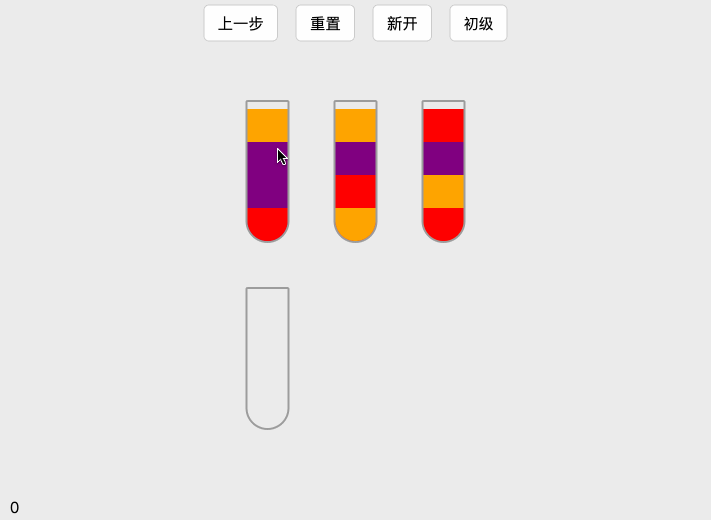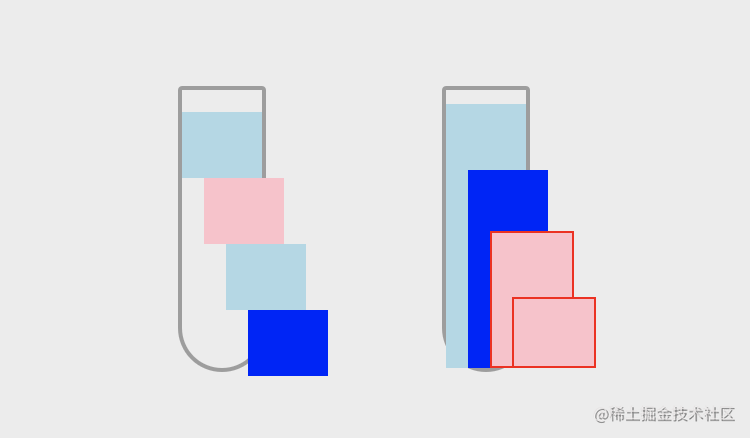# 摸鱼的时候玩一个小游戏吧。## 了解小游戏玩法

1. 随机创建四个瓶子并且随机的颜色块
2. 点击瓶子时把当前颜色块的最上一个放到另外一个瓶子的最上面
3. 如果当前的颜色大于2是一样的则叠加倒入到另一个瓶子的最上面
4. 可以返回上一步历史记录操作
5. 切换难度 0(普通), 1(中等), 2(困难), 3(非常难)
6. 记录多少步长

## 拆分需求内容

### 初始化操作

``````class ColorSort {
// 默认参数
static DEFAULT_OPTIONS = {
el: null, // 游戏的容器
level: 0, // 难度
num: 4  // 初始化的颜色块数量
}
constructor(options) {
this.options = Object.assign({}, ColorSort.DEFAULT_OPTIONS, options)
this.colorArr = []
this.init()
}
init() {
// 创建 DOM 元素渲染
this.options.el.innerHTML = `
<div class="color-sort__btns">
<div class="btn color-sort__prev">上一步</div>
<div class="btn color-sort__reset">重置</div>
<div class="btn color-sort__restart">新开</div>
<div class="btn color-sort__level">
<select class="color-sort__select">
<option value="0">初级</option>
<option value="1">中级</option>
<option value="2">困难</option>
<option value="3">特难</option>
</select>
</div>
</div>
<div class="color-sort__wrapper"></div>
<div class="color-sort__step">0</div>
`
// 创建随机颜色
this.colorArr = this.randomColorArr()
// 渲染界面
this.render()
}
}

### 获取随机颜色

``````// 重新渲染 colorArr 数组
randomColorArr() {
// 保存所有瓶子颜色的栈
let arr = []
// 根据等级创建多个瓶子，并且保证最后一个瓶子为空
for (let i = 0; i < this.options.level + 3; i++) {
const color = this._createEmpty()
arr = arr.concat(color)
}
// 打乱颜色
const colorShuffle = this._shuffle(arr)
// 重组颜色
const colorArr = this._rebuild(colorShuffle)
// 在后面添加一个空的瓶子
colorArr.push(this._createEmpty('transparent'))
​
return colorArr
}

``````// 打乱颜色
_shuffle(arr) {
// 复制一份数组
let _arr = arr.slice()
for (let i = 0; i < _arr.length; i++) {
let j = this._getRandomInt(0, i)
let t = _arr[i]
_arr[i] = _arr[j]
_arr[j] = t
}
return _arr
}
// 获取一个随机数，区间是 [min, max)
_getRandomInt(min, max) {
return Math.floor(Math.random() * (max - min + 1) + min)
}

``````// 重组颜色
_rebuild(arr) {
const result = []
const _arr = arr.slice()
const group = _arr.length / this.options.num
// 做一下数据切割，保证每个瓶子有 5 个颜色
for (let i = 0; i < group; i++) {
result.push(_arr.slice(i * this.options.num, (i + 1) * this.options.num))
}
return result
}

### 页面渲染

``````render() {
const colorArr = this.colorArr
// 一行有 3 个
const col = 3
// 用于计算的变量值
let index = 0
for (let i = 0; i < colorArr.length; i++) {
const calc = i % col
if (calc === 0 && i !== 0) {
index++
}
// 创建瓶子
const item = document.createElement('div')
item.className = 'color-sort__item'
item.style.height = `\${height}px`
// ...
}
}``````// 单个色块的 宽度
static WIDTH = 40
// 单个色块的 高度
static HEIGHT = 30
// 渲染
render() {
const colorArr = this.colorArr
const wrapper = this.options.el.querySelector('.color-sort__wrapper')
// 计算需要有多少列，如果超出颜色数组长度大于 6 就改成一行 4 个（纯粹是为了好看点）
const col = colorArr.length > 6 ? 4 : 3
// 单个瓶子的间距
const space = 40
// 单个瓶子上方的空白间隙
// 计算一个瓶子的高度
const height = this.options.num * ColorSort.HEIGHT + padding
// 用于计算的变量值
let index = 0
​
// 创建瓶子
for (let i = 0; i < colorArr.length; i++) {
const calc = i % col
if (calc === 0 && i !== 0) {
index++
}
// 创建一个瓶子
const item = document.createElement('div')
item.className = 'color-sort__item'
item.style.height = `\${height}px`
item.style.top = `\${(height * index) + (space * index)}px`
item.style.left = `\${(calc * ColorSort.WIDTH) + (calc * space)}px`
​
// 绑定点击事件
for (let j = 0; j < this.options.num; j++) {
const color = document.createElement('div')
color.className = 'color'
color.style.height = `\${(this.options.num - j) * ColorSort.HEIGHT}px`
color.style.zIndex = j
color.style.backgroundColor = colorArr[i][j]
item.appendChild(color)
}
wrapper.appendChild(item)
}
// 给父级元素添加宽高
wrapper.style.width = `\${ColorSort.WIDTH * col + (col - 1) * space}px`
wrapper.style.height = `\${height * (index + 1) + index * space}px`
// 最后插入到 dom 中
this.options.el.appendChild(wrapper)
// 结束动画状态
ColorSort.isMoving = false
}

``````.color-sort .color-sort__wrapper {
position: absolute;
top: 50%;
left: 50%;
transform: translate(-50%, -50%);
}

### 点击瓶子操作

1. 判断第二次点的瓶子是否满格（满格为 this.options.num 次），如果满格，置换成第一次点击
2. 第一次点击的最上面的瓶子内颜色应该， 加入到第二次点击的瓶子最上面的颜色中
3. 如果第一次点击的瓶子上面同时有两个或者两个以上的相同的颜色，则再第二次点击过程中把相同的颜色都倒入到下个瓶子上面
4. 点击之后数据改变，重新渲染操作
5. 保存历史记录
``````// 点击的次数，是否是第一次点击（为 0）或者第二次点击（为 1）
static flag = 0
static firstDom = null
static secondDom = null
static firstIndex = 0
static secondIndex = 0
static firstPostion = {}
​
// 点击事件
handleClick(dom, index) {
// 动画正在运行时不能点击
if (ColorSort.isMoving) return
// 第一次点击
if (ColorSort.flag === 0) {
// 如果点击的是空瓶子，则不做任何操作
let isOk = this.colorArr[index].find((item) => item !== 'transparent')
if (!isOk) {
return
}
this.setFirstCommon(dom, index)
}
// 第二次点击
else if (ColorSort.flag === 1) {
ColorSort.isMoving = true
​
ColorSort.secondIndex = index
ColorSort.flag = 0
ColorSort.secondDom = dom
​
ColorSort.firstDom.style.transform = 'scale(1)'
// ...
}
}
​
// 设置初始属性
setFirstCommon(dom, index) {
ColorSort.isMoving = false
​
ColorSort.firstIndex = index
ColorSort.flag = 1
ColorSort.firstDom = dom
// 保存第一次点击的位置
ColorSort.firstPostion = {
top: dom.offsetTop,
left: dom.offsetLeft,
}
​
ColorSort.firstDom.style.transform = 'scale(1.08)'
}

``````// 获取点击时瓶子内的最上层颜色（**关键代码**）
_getColor(arr) {
const obj = {
index: arr.length,
count: arr.length,
color: 'transparent'
}
for (let i = 0; i < arr.length;) {
if (arr[i] === 'transparent') {
i++
continue
}
let count = 0
for (let j = i; j < arr.length; j++) {
if (arr[i] === arr[j]) {
count++
} else {
break
}
}
obj.color = arr[i] // 透明色之后出现的第一个颜色值
obj.count = count // 连续出现相同颜色的次数
obj.index = i // 可以代表透明色之后出现的第一个颜色的索引，也可以代表透明的颜色的个数
break
}
return obj
}

``````// 取出第一次点击的数组
const firstArr = this.colorArr[ColorSort.firstIndex]
// 取出第二次点击的数组
const secondArr = this.colorArr[ColorSort.secondIndex]
​
// 第一次点击的数组下首个有颜色的色块的 索引，个数和颜色值
const firstColor = this._getColor(firstArr)
const secondColor = this._getColor(secondArr)
​
if (ColorSort.firstIndex === ColorSort.secondIndex) {
this.setFirstCommon(dom, index)
return
}
​
// 当第一次点击的颜色个数大于第二次点击的瓶子内的透明颜色个数时，判断是超出的，则重新设置成第一次点击的瓶子
if (firstColor.count > secondColor.index) {
this.setFirstCommon(dom, index)
return
}
​
// 先取出第二次点击的透明颜色的个数，即，等于第一次点击的相同颜色的个数
const transparentArr = secondArr.slice(0, firstColor.count)
​
const firstArrDel = firstArr.splice(
firstColor.index,
firstColor.count,
...transparentArr
)
​
secondArr.splice(
secondColor.index - firstColor.count,
firstColor.count,
...firstArrDel
)
​
// 给第一次的操作的数据修改
this.colorArr.splice(ColorSort.secondIndex, 1, firstArr)
// 给第二次的操作的数据修改
this.colorArr.splice(ColorSort.secondIndex, 1, secondArr)
​
// 添加动画
this.animate(firstColor, secondColor)

### 执行动画

• 第一，相同颜色的添加，就是第一次和第二次的瓶子内第一个颜色都相同
• 第二，不同颜色的添加
• 第三，添加到空瓶中

``````// 执行动画
animate(firstColor, secondColor) {
// 可以直接取第一个颜色作为动画的改变，因为不管怎样第一个颜色始终会是 transparent
// 设置第二次点击瓶子中第一个颜色的初始高度
const secondColorsDom = ColorSort.secondDom.querySelectorAll('.color')
// 设置第二个颜色的初始高度
secondColorsDom.style.height = 0
​
ColorSort.firstDom.style.zIndex = 100
ColorSort.firstDom.style.top = `\${ColorSort.secondDom.offsetTop - 90}px`
ColorSort.firstDom.style.left = `\${ColorSort.secondDom.offsetLeft - 70}px`
ColorSort.firstDom.style.transform = 'rotate(75deg)'
​
setTimeout(() => {
// 获取点击第一次下面的全部色块
const firstColorsDom = ColorSort.firstDom.querySelectorAll('.color')
// 如果超过 1 个 就没有后面的相同，超过一个就去除掉
// 去掉除第一个颜色下所有相同的颜色
if (firstColor.count > 1) {
const j = firstColor.index + firstColor.count - 1
for (let i = firstColor.index; i <= j; i++) {
if (i !== firstColor.index) {
}
}
}
// 给其添加样式，让高度为 0
​
// 设置动画色块的初始颜色
secondColorsDom.style.backgroundColor = firstColor.color
// 开始加入动画（向上溢出），实际高度 = （第一次点击的颜色个数 + 瓶子总格数 - 第二次点击的颜色索引）* 色块高度
secondColorsDom.style.height = `\${(firstColor.count + (this.options.num - secondColor.index)) * ColorSort.HEIGHT}px`
}, 800)
​
// 监听动画完成
setTimeout(this.listenAnimate.bind(this), 1200)
}

### 添加历史记录

``````// 添加历史记录
ColorSort.history.push(JSON.parse(JSON.stringify(this.colorArr)))
// 如果历史记录超过 10 条，则删除最早的一条
if (ColorSort.history.length > ColorSort.MAX_HISTORY_LENGTH) {
ColorSort.history.splice(0, 1)
}
}
// 撤销
prevHistory() {
if (ColorSort.history.length === 1) {
return
}
ColorSort.history.pop()
const color = ColorSort.history[ColorSort.history.length - 1]
this.colorArr = JSON.parse(JSON.stringify(color))
this.render()
}